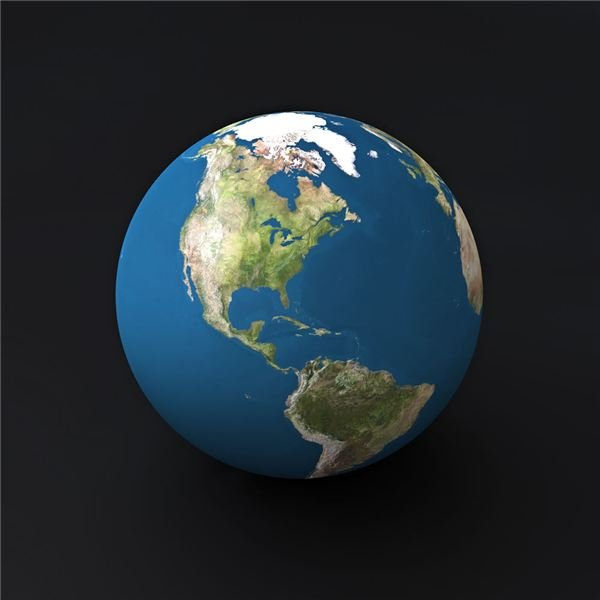The Equator and the Shape of the Earth

Prelab Prep and Discussion

Have students read the entire science activity and then discuss the following:

• Why do we use models when studying Earth Science?
• How does the model that you will build differ in mass, size, and spin velocity than the rotating Earth?

Materials needed:

• long, sharpened pencil
• construction paper
• ruler
• scissors
• tape
• hole punch
• sheet of paper
• observation table

Procedure

1. Using the construction paper, cut 2 separate strips that each measure 1 inch by 16 inches.
2. Mark an X at the center of each strip.
3. Cross the strips at their centers, and tape them together.
4. Bring the four loose ends together. Overlap the ends and secure together with tape.
5. Use a hole punch to make a hole in the center of each end where the strips overlap.
6. Push the pencil through the holes in each end.
7. Hold each end of the pencil and roll the pencil in your fingers. Observe what happens to the equator and the poles.
8. Record your observations of the equator and poles in your observation table under Observation 1.
9. Re-form a sphere with the strips of paper.
10. Place a sheet of paper on a flat surface.
11. Place the tip of the pencil on the paper. Twirl the pencil. Observe the equator and the poles. Record your observations in the observation table under Observation 2.

Analysis

1. What does the sphere represent? (The sphere represents the Earth.)2. What does the rolling or twirling of the pencil represent? (Rolling or twirling the pencil represents the rotation of Earth on its axis.)
3. Describe what happens at the equator when the pencil is rolled or twirled. (The center bulges out.)
4. Describe what happens at the poles when the pencil is rolled or twirled. (The paper near the ends of the pencil slides toward the center.)
5. How is this model different from Earth? Explain. (Answers may vary. The model is not solid and has far less mass than Earth. The material making up the model is uniform compared to the Earth.)

Conclusion and Extension

Write a short paragraph describing how your Earth model is similar to what happens as Earth rotates.

How can you find out how much the sphere in your model flattened? Design an experiment to figure out how much your sphere flattens from its original shape when it is rolled.